https://doi.org/10.5194/ms-13-899-2022
https://doi.org/10.5194/ms-13-899-2022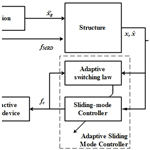# Adaptive sliding-mode control for improved vibration mitigation in civil engineering structures

Khaled Zizouni, Abdelkrim Saidi, Leyla Fali, Ismail Khalil Bousserhane, and Mohamed Djermane
Abstract

Seismic vibration control using a magneto-rheological damper is a technique that interests several researchers around the world. This technique offers suitable structural building protection, ensuring human safety against earthquake excitation damages. The robustness of these devices depended in many cases on the designed law of control. Over the years, research focused on the development and modelling of various controllers to enhance the structural vibration elimination of buildings. The emphasis of this paper is on the evaluation of semi-active control robustness to reduce the displacements of a three-storey tested structure. The semi-active control device is a magneto-rheological fluid damper installed on the ground floor of the earthquake's excited structure and is controlled by an adaptive non-linear controller coupled to a clipped optimal algorithm to drive the current. The proposed controller is a sliding-mode controller reinforced by an adaptive technique to perform the control gain choice and overcome the chattering problem. The present law of adaptation is a switching conditional law between two laws offering the required gain depending on the system state. The numerical simulation results prove the effectiveness of the proposed semi-active control strategy in attenuating the displacements of the tested structure.

Share
Dates
1 Introduction

Earthquake excitations cause building structure damage, city destruction, and human deaths. They directly affect buildings or their structural components through the brutal motion of the ground-propagating vibration. During the last century, earthquakes caused deaths and homelessness of millions of people and destroyed several cities. Algerian towns were affected by strong earthquakes during this period: Algiers in 1916, El Asnam in 1980, Constantine in 1985, Tipasa in 1989, Mascara in 1994, Algiers in 1996, and Boumerdès in 2003 . The two strongest earthquakes in Algeria were the El Asnam earthquake of 10 October 1980 with a magnitude of 7.2 that killed about 2500 people and the Boumerdès earthquake of 21 May 2003 with a magnitude of 6.8 that killed 2278 people . The need for a developed technology that can easily and in real time minimize structural vibrations caused by earthquake excitation became an extreme obligation. During the last decade, the control of structures has emerged as a possible technological solution for structural vibration reduction, in which a control device is introduced to the main structure to modify and decrease the damping. The control can be an active, semi-active, or passive one according to the used devices.

The passive control system is based principally on isolation or energy dissipation devices designed to develop an opposite control force to the motion of the structure induced by earthquake excitation. The greatest advantages of these devices are that they are simple, economic, and do not need energy to operate, but they have limited robustness against strong dynamical loads, which limits their utilization. The passive control technologies have been widely employed for a large number of buildings in the world. In a seismic isolation with a lead rubber bearing isolator of nuclear Japanese reactor buildings is evaluated. The isolated reactor was exposed to a series of seismic responses to discuss the feasibility of the proposed passive isolation system. proposed and analysed a seismic base isolation with a bearing isolation exposed to an earthquake excitation. The active control system is more expansive than the passive one: it consists of sensors to measure structural responses, a process to treat responses and to compute the required control force with an adequate control law, and actuators to produce the control force and energy source to power the actuators. These systems use in real time a high external energy to modify the undesirable dynamic behaviour of the structure. The measured responses of the excited structures by sensors are sent to the unit treatment in which the required control force is calculated using a control strategy. From the calculated control force, the applied force is determined, and the actuator receives information from unit treatment and provides this force to the structure.

Recently, major attention has been paid to active structural control research. investigated the structural vibration reduction of a 40-storey structure subjected to earthquake excitation with a soil–structure interaction effect. The active control of the structure is ensured by an active tuned mass damper controlled with two linear controllers. The first proposed controller was the proportional integral derivative controller (PID), while the second proposed controller was a linear quadratic regulator (LQR) and the parameters of the damper were optimized using a particle swarm optimization . Semi-active control is considered to be the system combining the best features of passive and active systems. It offers the adaptability characteristics of an active control system without requiring large power sources and the stability characteristics of passive control systems. This system can modify mechanical properties using only a computer and a small electronic device with a low power source to operate as a battery, which is critical during excitation events. Numerous studies have been performed on the investigation of the application of semi-active control in structure protection against earthquakes or wind excitation. A semi-active control is designed to improve the seismic behavior of a three-storey excited structure in Bozorgvar and Zahrai (2019) using a magneto-rheological damper controlled by an adaptive neuro-fuzzy where a genetic algorithm adjusts premise and subsequent parameters. The results of the proposed algorithm are compared to the results of the neural network predictive control algorithm and linear quadratic Gaussian and clipped optimal control applied to the same case. The evaluation criteria show the effectiveness of the proposed control in reducing structural vibrations subjected to earthquake excitation.

The hybrid control system can also be considered one of the structural control systems which are a combination of the aforementioned systems. Generally, the hybrid system is composed of an active system combined with a passive system. Thereby, the hybrid system offers the advantages of both coupled systems. Remarkable searches have been made over the last decade. An eight-storey structure is controlled using a hybrid control composed of viscous liquid dampers as a passive control and an active tuned mass damper. The active tuned mass damper is installed on the top floor, and on each one of the other floors a viscous liquid damper is placed. To prove the effectiveness of the proposed hybrid control system in eliminating structural vibrations, this structure was subjected to the Kobe, El Centro, Hachinohe, and Northridge earthquakes .

However, in order to tune the control force of the active or semi-active control device in real time, the design of a robust controller is considered an essential step. In the existing literature, several control techniques and algorithms are quoted. Many papers have investigated the use of non-linear controllers in vibration control. In , an adaptive back-stepping control is proposed to control a 10-storey building using a magneto-rheological damper coupled with passive base isolation. Moreover, a sliding-mode controller was designed to control a multi-freedom structure using a magneto-rheological damper in an experimental and simulated study . From a theoretical point of view, linear controllers are the most preferred one in structural vibration control. These controllers are simple, easy to implement, and converge quickly. Despite this, these controllers do not operate in the presence of non-linearities or uncertainties in the controlled model, which is the practical case. proposed a linear quadratic regulator to control a scaled three-storey structure subjected to the 1940 El Centro earthquake records. The numerical simulation results show the robustness of the linear proposed controller coupled with a clipped optimal algorithm to adjust the magneto-rheological damper tension . Another class of non-linear controllers is called the intelligent controllers, in which the designs do not need any mathematical model. A neural network algorithm is proposed in based on an LQR control trainer to suppress the vibration of civil engineering excited structures using a magneto-rheological damper. The intelligent controller is composed of four layers and three neurons in each hidden layer. The performed neural network controller used only two inputs, in contrast to the original controller needing six inputs for a three-storey scaled structure, reducing the number of sensors to about 77 %. The proposed controller robustness is proven by the numerical simulation responses of a three-storey scaled structure exposed to both the 2011 Tohoku and 2003 Boumerdès earthquakes.

In this paper, an adaptive non-linear controller is proposed to reduce undesirable vibrations of a scaled structure caused by seismic excitation. The controller is designed to control a semi-active magneto-rheological damper coupled to a clipped algorithm current driver. The sliding-mode controller is reinforced by a switching adaptive law to ensure stability and to suppress the chattering phenomenon. The suggested law offers adaptation of the boundary layer thickness in the sliding surface of the controller. Therefore, the switching jump length is adapted to maintain the desired stability of the system, although the proposed adaptation law switches between two laws which calculated the gain of control depending on the system position compared to the sliding surface. Under the 1940 El Centro earthquake record, the effectiveness of the proposed semi-active control strategy is evaluated. In order to prove the robustness of the control, the numerical simulation results of the controlled and uncontrolled structures are compared and discussed. The rest of the paper is organized as follows: Sect. 2 is reserved for the system presentation in which the magneto-rheological damper and the test scaled structure modelling are illustrated, where the mathematical model of the controlled system is explained. Next, in Sect. 3 the controller and the adaptive law are formulated, ensuring the Lyapunov stability criterion. The numerical example of the 1940 El Centro earthquake is presented and the comparison of the results of the two cited cases is shown in Sect. 4. Finally, this paper is concluded by the drawn conclusion in the last section.

2 The system presentation

## 2.1 The magneto-rheological damper model

The magneto-rheological device is considered one of the interesting semi-active control devices. The properties offered by the magneto-rheological fluid attracted several numerical and experimental studies and investigations. The main investigations focused on the mathematical modellization of the high non-linear behaviour of the controllable fluid. Nonetheless, in the presence of the current field, this fluid can change its physical properties in milliseconds only. In addition, the device offered simplicity, reliability, and suitable stability in temperature variation . Recently, magneto-rheological dampers have been used to attenuate structural vibrations caused by earthquake excitation in several buildings. The semi-active control device is presented in Fig. 1.

Therefore, from the invention of the magneto-rheological fluid, several studies presented mathematical modelling for the non-linear behaviour of this fluid . The literature cited different proposed mathematical models, such as the Bingham model (Bingham1917), the augmented Bingham model , the Herschel–Bulkley model , the Bouc model (Bouc1971), and the Bouc–Wen model (Wen1976), although the most recently used model is the one established by called the generalized Bouc–Wen model presented in Fig. 2.

The mathematical generalized Bouc–Wen model is described by the following equations:

$\begin{array}{}\text{(1)}& {c}_{\mathrm{1}}\stackrel{\mathrm{˙}}{y}={c}_{\mathrm{0}}\left(\stackrel{\mathrm{˙}}{x}-\stackrel{\mathrm{˙}}{y}\right)+{k}_{\mathrm{0}}\left(x-y\right)+\mathit{\alpha }z,\text{(2)}& \stackrel{\mathrm{˙}}{z}=-\mathit{\gamma }|\stackrel{\mathrm{˙}}{x}-\stackrel{\mathrm{˙}}{y}|z|z{|}^{n-\mathrm{1}}-\mathit{\beta }\left(\stackrel{\mathrm{˙}}{x}-\stackrel{\mathrm{˙}}{y}\right)|z{|}^{n}+A\left(\stackrel{\mathrm{˙}}{x}-\stackrel{\mathrm{˙}}{y}\right),\text{(3)}& {f}_{\mathrm{MRD}}={c}_{\mathrm{0}}\left(\stackrel{\mathrm{˙}}{x}-\stackrel{\mathrm{˙}}{y}\right)+{k}_{\mathrm{0}}\left(x-y\right)+{k}_{\mathrm{1}}\left(x-{x}_{\mathrm{0}}\right)+\mathit{\alpha }z,\text{(4)}& \mathit{\alpha }={\mathit{\alpha }}_{a}+{\mathit{\alpha }}_{b}u,\text{(5)}& {c}_{\mathrm{1}}={c}_{\mathrm{1}a}+{c}_{\mathrm{1}b}u,\text{(6)}& {c}_{\mathrm{0}}={c}_{\mathrm{0}a}+{c}_{\mathrm{0}b}u,\text{(7)}& \stackrel{\mathrm{˙}}{u}=-\mathit{\eta }\left(u-v\right),\end{array}$

where x and $\stackrel{\mathrm{˙}}{x}$ are the damper's rod displacement and velocity, k0 and c0 and k1 and c1 are, respectively, the accumulator stiffness and viscous damping at low velocity and the accumulator stiffness and viscous damping at high velocity, z is the hysteresis behaviour variable, fMRD is the damper-generated force, A, α, β, n, and γ are the parameters of the model shape, x0 is the initial deflection of the spring, y is the damper displacement, u, v, and η are the dynamic phenomenological variable, the applied voltage, and the time response factor, and c1a, c1b, c0a, c0b, αa, and αb are the magneto-rheological damper parameters depending on the applied voltage.

The parameter values of the augmented Bouc–Wen model are shown in Table 1 .

To show the typical hysteresis behaviour of the MR damper, a sinusoidal displacement is applied to the device with a frequency of 2.5 Hz and 1.5 cm amplitude. By contrast, a different constant voltage level is associated with this sinusoidal displacement. The chosen voltage levels are 0, 0.5, 0.75, and 1 V, respectively.

The generated force of the MR damper function of time is illustrated in Fig. 3. Thus, the hysteresis behaviour of the device is depicted in Figs. 4 and 5.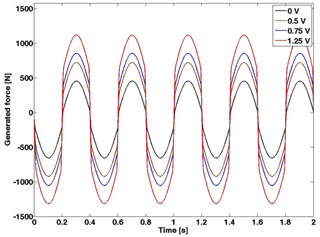Figure 3Generated force of the simulated Bouc–Wen model under sinusoidal excitation.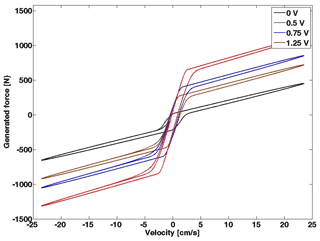Figure 4Force–displacement hysteresis loop of the simulated Bouc–Wen model under sinusoidal excitation.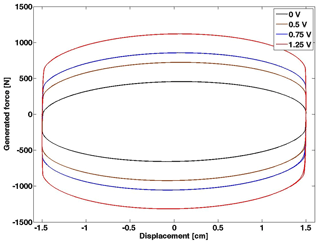Figure 5Force–velocity hysteresis loop of the simulated Bouc–Wen model under sinusoidal excitation.

## 2.2 The tested scaled structure model

The proposed semi-active control is evaluated using a three-storey scaled structure subjected to a ground motion excitation ${\stackrel{\mathrm{¨}}{x}}_{\mathrm{g}}$. The semi-active control device is a magneto-rheological damper installed on the first floor of the tested structure presented in Fig. 6.

The dynamic equation of motion of the system in Fig. 6 is written as

$\begin{array}{}\text{(8)}& M\stackrel{\mathrm{¨}}{x}+C\stackrel{\mathrm{˙}}{x}+Kx=M\mathrm{\Lambda }{\stackrel{\mathrm{¨}}{x}}_{\mathrm{g}}+\mathrm{\Gamma }{f}_{\mathrm{c}},\end{array}$

where x, $\stackrel{\mathrm{˙}}{x}$, and $\stackrel{\mathrm{¨}}{x}$ are the displacement, velocity, and acceleration vectors of the structure, and M, C, and K are the mass, damping, and stiffness matrices of the structure defined as follows.

$\begin{array}{}\text{(9)}& M=\left[\begin{array}{ccc}{m}_{\mathrm{1}}& \mathrm{0}& \mathrm{0}\\ \mathrm{0}& {m}_{\mathrm{2}}& \mathrm{0}\\ \mathrm{0}& \mathrm{0}& {m}_{\mathrm{3}}\end{array}\right]=\left[\begin{array}{ccc}\mathrm{98.3}& \mathrm{0}& \mathrm{0}\\ \mathrm{0}& \mathrm{98.3}& \mathrm{0}\\ \mathrm{0}& \mathrm{0}& \mathrm{98.3}\end{array}\right]\phantom{\rule{0.25em}{0ex}}\left(\mathrm{kg}\right)\text{(10)}& \begin{array}{rl}C& =\left[\begin{array}{ccc}{c}_{\mathrm{1}}+{c}_{\mathrm{2}}& -{c}_{\mathrm{2}}& \mathrm{0}\\ -{c}_{\mathrm{2}}& {c}_{\mathrm{2}}+{c}_{\mathrm{3}}& -{c}_{\mathrm{3}}\\ \mathrm{0}& -{c}_{\mathrm{3}}& {c}_{\mathrm{3}}\end{array}\right]\\ & =\left[\begin{array}{ccc}\mathrm{175}& -\mathrm{50}& \mathrm{0}\\ -\mathrm{50}& \mathrm{100}& -\mathrm{50}\\ \mathrm{0}& -\mathrm{50}& \mathrm{50}\end{array}\right]\phantom{\rule{0.25em}{0ex}}\left(\mathrm{N}\phantom{\rule{0.125em}{0ex}}\mathrm{s}\phantom{\rule{0.125em}{0ex}}{\mathrm{m}}^{-\mathrm{1}}\right)\end{array}\end{array}$

$\begin{array}{}\text{(11)}& \begin{array}{rl}K& =\left[\begin{array}{ccc}{k}_{\mathrm{1}}+{k}_{\mathrm{2}}& -{k}_{\mathrm{2}}& \mathrm{0}\\ -{k}_{\mathrm{2}}& {k}_{\mathrm{2}}+{k}_{\mathrm{3}}& -{k}_{\mathrm{3}}\\ \mathrm{0}& -{k}_{\mathrm{3}}& {k}_{\mathrm{3}}\end{array}\right]\\ & ={\mathrm{10}}^{\mathrm{5}}\left[\begin{array}{ccc}\mathrm{12}& -\mathrm{6.84}& \mathrm{0}\\ -\mathrm{6.84}& \mathrm{13.7}& -\mathrm{6.84}\\ \mathrm{0}& -\mathrm{6.84}& \mathrm{6.84}\end{array}\right]\phantom{\rule{0.25em}{0ex}}\left(\mathrm{N}\phantom{\rule{0.125em}{0ex}}{\mathrm{m}}^{-\mathrm{1}}\right)\end{array}\end{array}$

fc is the control force, Λ and Γ are the earthquake effect vectors, and the damper position vector is defined as follows.

$\begin{array}{}\text{(12)}& \mathrm{\Lambda }={\left[\begin{array}{ccc}\mathrm{1}& \mathrm{1}& \mathrm{1}\end{array}\right]}^{T}\text{(13)}& \mathrm{\Gamma }={\left[\begin{array}{ccc}-\mathrm{1}& \mathrm{0}& \mathrm{0}\end{array}\right]}^{T}\end{array}$
3 Semi-active control algorithm

Since its appearance in the 1950s the sliding-mode controller has been considered one of the most interesting non-linear controllers. The robustness of this controller, especially in the presence of the uncertainties or the system condition variations, makes it one of the most used controllers. However, several investigations and studies are interested in structural vibration control using a sliding-mode controller, whereas the high-frequency oscillation of the switch controller part caused the chattering problem which can affect the system stability and precision .

Therefore, various solutions were investigated to suppress the undesirable chattering effect and to perform the sliding-mode controller. Furthermore, several tested techniques proved their efficiency as the boundary layer technique using the saturation function , the high-order sliding mode , the fuzzy sliding mode , the neural network sliding mode, the adaptive sliding mode , and others .

Consider the non-linear system

$\begin{array}{}\text{(14)}& \stackrel{\mathrm{˙}}{x}=f\left(x,t\right)+g\left(x,t\right)u.\end{array}$

x and u are, respectively, the state vector and the control input, and f(x) and g(x) are smooth functions.

The sliding-mode controller (SMC) is composed of two components as follows:

$\begin{array}{}\text{(15)}& {u}_{\mathrm{SMC}}={u}_{\mathrm{eq}}+{u}_{\mathrm{s}}.\end{array}$

ueq is the equivalent part of the controller and us is the controller switching part given as

$\begin{array}{}\text{(16)}& {u}_{\mathrm{eq}}\left(x,t\right)=-\left(s\left(x,t\right)g\left(x,t\right){\right)}^{-\mathrm{1}}s\left(x\right)f\left(x,t\right),\text{(17)}& {u}_{\mathrm{s}}=G\cdot \mathrm{sgn}\left(s\left(x,t\right)\right),\end{array}$

where G is the control switch gain, sgn is the signum function, and s is the sliding surface given by

$\begin{array}{}\text{(18)}& s\left(x,t\right)=Se.\end{array}$

S is the defined positive switching matrix of the sliding surface and e is the tracking error of the state vector.

In this stage, the switching part of the sliding-mode controller in Eq. (17) caused finite frequency in the dynamic sliding motion called chattering. In this paper, a switching law adaptation is proposed to overcome the chattering problem and perform the switching part of the sliding-mode controller. The main goal of adaptive control is to design the system to maintain the same properties under uncertain conditions. Thereby, the controller modifies its law to manage the new situation based on the currently collected information. Thus, Eq. (17) becomes

$\begin{array}{}\text{(19)}& {u}_{\mathrm{as}}=\stackrel{\mathrm{̃}}{G}\cdot \mathrm{sgn}\left(s\left(x,t\right)\right).\end{array}$

uas is the adaptive controller switching part and $\stackrel{\mathrm{̃}}{G}$ is the adapting gain depending on the following switching law :

$\begin{array}{}\text{(20)}& \begin{array}{rl}& \mathrm{If}\phantom{\rule{0.25em}{0ex}}\phantom{\rule{0.25em}{0ex}}|s\left(x,t\right)|>\mathrm{0}⟹\stackrel{\mathrm{˙}}{\stackrel{\mathrm{̃}}{G}}=\stackrel{\mathrm{‾}}{{G}_{\mathrm{1}}}\cdot |\left(s\left(x,t\right)|\\ & \phantom{\rule{1em}{0ex}}\mathrm{with}\phantom{\rule{0.25em}{0ex}}\phantom{\rule{0.25em}{0ex}}\stackrel{\mathrm{‾}}{{G}_{\mathrm{1}}}>\mathrm{0}\phantom{\rule{0.25em}{0ex}}\phantom{\rule{0.25em}{0ex}}\mathrm{and}\phantom{\rule{0.25em}{0ex}}\phantom{\rule{0.25em}{0ex}}\stackrel{\mathrm{̃}}{G}\left(\mathrm{0}\right)=\mathrm{0},\end{array}\text{(21)}& \mathrm{else}\phantom{\rule{0.25em}{0ex}}\phantom{\rule{0.25em}{0ex}}\stackrel{\mathrm{̃}}{G}=\stackrel{\mathrm{‾}}{{G}_{\mathrm{2}}}\cdot |\mathit{\rho }|+\stackrel{\mathrm{‾}}{{G}_{\mathrm{3}}}\phantom{\rule{0.25em}{0ex}}\phantom{\rule{0.25em}{0ex}}\mathrm{with}\phantom{\rule{0.25em}{0ex}}\phantom{\rule{0.25em}{0ex}}\mathit{\tau }\cdot \stackrel{\mathrm{˙}}{\mathit{\rho }}+\mathit{\rho }=\mathrm{sgn}\left(s\left(x,t\right)\right).\end{array}$

where τ is a positive time constant, ρ is the average value of the low-pass filter; $\stackrel{\mathrm{‾}}{{G}_{\mathrm{1}}}$ and $\stackrel{\mathrm{‾}}{{G}_{\mathrm{2}}}$ are positive gains. The Lyapunov stability is satisfied if the following condition is satisfied:

$\begin{array}{}\text{(22)}& \stackrel{\mathrm{˙}}{s}\left(x,t\right)\cdot s\left(x,t\right)\le \mathrm{0}.\end{array}$

For this reason, the first Lyapunov candidate function of Eq. (20) is proposed as

$\begin{array}{}\text{(23)}& {V}_{\mathrm{1}}=\frac{\mathrm{1}}{\mathrm{2}}{s}^{\mathrm{2}}+\frac{\mathrm{1}}{\mathrm{2}\mathit{\gamma }}\left(G-{G}^{*}{\right)}^{\mathrm{2}},\text{(24)}& \begin{array}{rl}{\stackrel{\mathrm{˙}}{V}}_{\mathrm{1}}& =s\cdot \mathit{\chi }\left(x,t\right)-s\cdot \mathit{\varphi }\left(x,t\right)\cdot G\cdot \mathrm{sgn}\left(s\right)\\ & +\frac{\mathrm{1}}{\mathit{\gamma }}\left(G-{G}^{*}\right){\stackrel{\mathrm{‾}}{G}}_{\mathrm{1}}\cdot |s|,\end{array}\end{array}$

where G* is the limit value of G, and $\mathit{\chi }\left(x,t\right)=\frac{\partial s}{\partial t}\frac{\partial s}{\partial x}f\left(x,t\right)$ and $\mathit{\varphi }\left(x,t\right)=\frac{\partial s}{\partial x}g\left(x,t\right)$ are unknown functions limited by the values χm, ϕm, and ϕM as $|\mathit{\chi }\left(x,t\right)|\le {\mathit{\chi }}_{m}$ and ${\mathit{\varphi }}_{m}\le \mathit{\varphi }\left(x,t\right)\le {\mathit{\varphi }}_{M}$, where ϕ(x,t) is assumed to be positive.

$\begin{array}{}\text{(25)}& {\stackrel{\mathrm{˙}}{V}}_{\mathrm{1}}\le |s|\cdot {\mathit{\chi }}_{m}-{\mathit{\varphi }}_{m}\cdot G|s|+\frac{\mathrm{1}}{\mathit{\gamma }}\left(G-{G}^{*}\right){\stackrel{\mathrm{‾}}{G}}_{\mathrm{1}}\cdot |s|,\text{(26)}& \begin{array}{rl}{\stackrel{\mathrm{˙}}{V}}_{\mathrm{1}}& \le |s|\cdot {\mathit{\chi }}_{m}-{\mathit{\varphi }}_{m}\cdot G|s|+{\mathit{\varphi }}_{m}\cdot {G}^{*}|s|-{\mathit{\varphi }}_{m}\cdot {G}^{*}|s|\\ & +\frac{\mathrm{1}}{\mathit{\gamma }}\left(G-{G}^{*}\right){\stackrel{\mathrm{‾}}{G}}_{\mathrm{1}}\cdot |s|,\end{array}\text{(27)}& \begin{array}{rl}{\stackrel{\mathrm{˙}}{V}}_{\mathrm{1}}& \le \left({\mathit{\chi }}_{m}-{\mathit{\varphi }}_{m}\cdot {G}^{*}\right)|s|+\left(-{\mathit{\varphi }}_{m}|s|+\frac{{\stackrel{\mathrm{‾}}{G}}_{\mathrm{1}}}{\mathit{\gamma }}|s|\right)\\ & \cdot \left(G-{G}^{*}\right),\end{array}\end{array}$

where ${\stackrel{\mathrm{˙}}{V}}_{\mathrm{1}}\cdot {V}_{\mathrm{1}}\le \mathrm{0}$ if ${G}^{*}>\frac{{\mathit{\chi }}_{m}}{{\mathit{\varphi }}_{m}}$ and $\mathit{\gamma }<\frac{{\stackrel{\mathrm{‾}}{G}}_{\mathrm{1}}}{{\mathit{\varphi }}_{m}}$ are satisfied.

The second Lyapunov candidate function of Eq. (21) is proposed as

$\begin{array}{}\text{(28)}& {V}_{\mathrm{2}}=\frac{\mathrm{1}}{\mathrm{2}}{s}^{\mathrm{2}}-\left({G}_{\mathrm{0}}|\mathit{\rho }|+\mathit{\delta }\right),\text{(29)}& {\stackrel{\mathrm{˙}}{V}}_{\mathrm{2}}=s\cdot \mathit{\chi }\left(x,t\right)-\left({G}_{\mathrm{0}}|\mathit{\rho }|+\mathit{\delta }\right),\end{array}$

where G0 is the positive gain, ρ is the average sgn(s) value, and the Laplace transformation of the first-order filter $\mathit{\tau }\cdot \stackrel{\mathrm{˙}}{\mathit{\rho }}+\mathit{\rho }=\mathrm{sgn}\left(s\right)$ is $\frac{\mathrm{1}}{\mathit{\rho }p+\mathrm{1}}$.

$\begin{array}{}\text{(30)}& {\stackrel{\mathrm{˙}}{V}}_{\mathrm{2}}=\mathit{\chi }\left(x,t\right)-\mathit{\rho }\cdot \left({G}_{\mathrm{0}}|\mathit{\rho }|+\mathit{\delta }\right)\end{array}$

${\stackrel{\mathrm{˙}}{V}}_{\mathrm{2}}\cdot {V}_{\mathrm{2}}\le \mathrm{0}$ is satisfied if $\mathit{\rho }=\left(\mathit{\delta }±\frac{\sqrt{{\mathit{\delta }}^{\mathrm{2}}±\mathrm{4}{G}_{\mathrm{0}}\cdot \mathit{\chi }\left(x,t\right)}}{\mathrm{2}{G}_{\mathrm{0}}}\right)$.

Therefore, the schematic block of the proposed semi-active adaptive control is illustrated in Fig. 7.

When x, $\stackrel{\mathrm{˙}}{x}$ are the structural responses, fMRD, fc are the magneto-rheological damper-generated force and the controller-calculated force. However, the magneto-rheological damper cannot activate the calculated force directly because the device operates based on the applied current voltage, and thus an algorithm is designed to control the current driver proportionately to the controller's calculated force. Hence, an off–on switching algorithm based on the Heaviside step function is proposed.

$\begin{array}{}\text{(31)}& v={v}_{max}\cdot H\left(\left({f}_{\mathrm{c}}-{f}_{\mathrm{MRD}}\right){f}_{\mathrm{MRD}}\right)\end{array}$

v is the required voltage, vmax is the maximum voltage of the current driver, and H(.) is the Heaviside step function.

4 Numerical example

To prove the robustness of the proposed semi-active control strategy using the magneto-rheological damper controlled by an adaptive sliding-mode controller, a numerical test is proposed. Based on the Matlab/Simulink software, the results were carried out and plotted. The tested scaled structure of Fig. 6 is subjected to a time-scaled earthquake record of the 1940 El Centro earthquake presented in Fig. 8.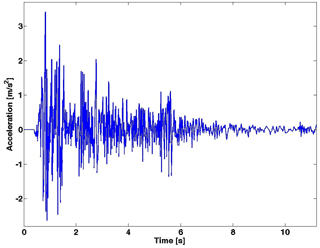Figure 8The NS (north–south) time-scaled component of the 1940 El Centro earthquake.

The numerical displacement results of the compared two cases of controlled and uncontrolled structures under the 1940 El Centro excitation are clearly shown for the three floors in Fig. 9. However, the acceleration-compared responses of the structure floors of the uncontrolled and controlled structures are depicted in Fig. 10.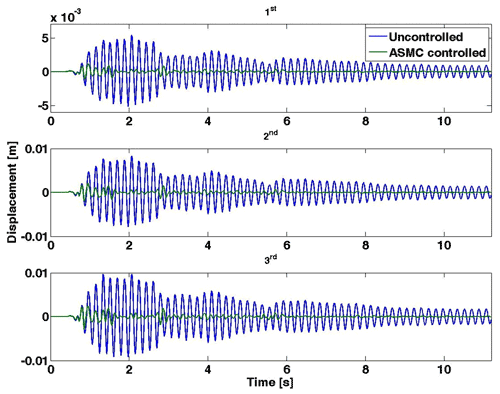Figure 9Displacement responses of the first, second, and third floors to the 1940 El Centro earthquake.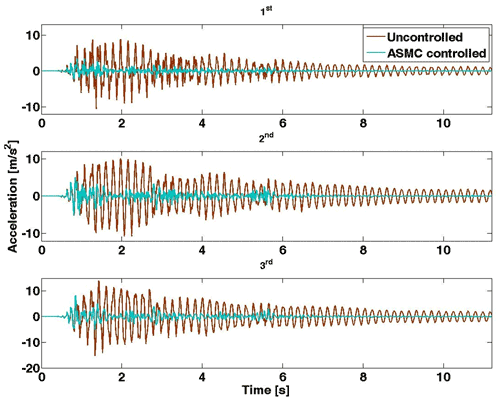Figure 10Structure floor acceleration responses to the 1940 El Centro earthquake.

Nevertheless, the time adjustment of the adaptive gain of Eqs. (20) and (21) under the 1940 El Centro earthquake is presented in Fig. 11. In addition, the response of the generated voltage to the magneto-rheological damper function of time during the 1940 El Centro earthquake is presented in Fig. 12.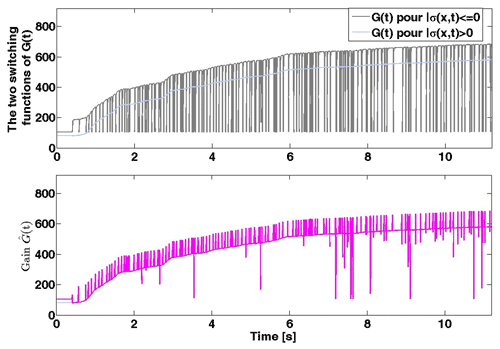Figure 11Time response of adaptive control gain under the 1940 El Centro earthquake.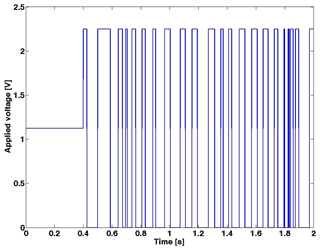Figure 12Zoomed time response voltages applied to the MR damper during the 1940 El Centro earthquake.

The effectiveness of the proposed adaptive semi-active control strategy is proven using the displacement floor reduction ratio given by

$\begin{array}{}\text{(32)}& {\mathrm{Rd}}_{\mathrm{r}}=\frac{|{x}_{i}^{max}|-max|{x}_{i}|}{|{x}_{i}^{max}|}.\end{array}$

Table 2The peak displacement reduction ratio of the SMC and ASMC during the 1940 El Centro earthquake.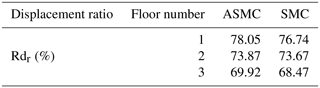Table 3Values of performance indices of the SMC and ASMC during the 1940 El Centro earthquake.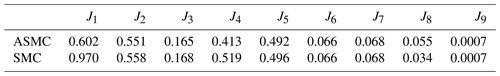In addition, two groups of evaluation indices are calculated to confirm the robustness of the semi-active adaptive sliding-mode controller in suppressing structural vibrations. The first group of the index (J1J6) is meant to appraise the structure responses under the proposed control and the second (J7J9) to evaluate the semi-active device performance under the adaptive controller. Hence, the performance indices are calculated as follows.

$\begin{array}{}\text{(33)}& {J}_{\mathrm{1}}=\frac{max|{d}_{\mathrm{i}}|}{{d}_{\mathrm{i}}^{max}}\text{(34)}& {J}_{\mathrm{2}}=\frac{max|{\stackrel{\mathrm{¨}}{x}}_{ai}|}{{\stackrel{\mathrm{¨}}{x}}_{ai}^{max}}\text{(35)}& {J}_{\mathrm{3}}=\frac{max|\sum {m}_{i}{\stackrel{\mathrm{¨}}{x}}_{ai}|}{{F}_{\mathrm{b}}^{max}}\text{(36)}& {J}_{\mathrm{4}}=\frac{max||{d}_{\mathrm{i}}||}{||{d}_{\mathrm{i}}^{max}||}\text{(37)}& {J}_{\mathrm{5}}=\frac{max\phantom{\rule{0.25em}{0ex}}{\stackrel{\mathrm{¨}}{x}}_{ai}}{{\stackrel{\mathrm{¨}}{x}}_{ai}^{max}}\text{(38)}& {J}_{\mathrm{6}}=\frac{max\sum {m}_{i}{\stackrel{\mathrm{¨}}{x}}_{ai}}{{F}_{\mathrm{b}}^{max}}\text{(39)}& {J}_{\mathrm{7}}=\frac{max|{f}_{\mathrm{MRD}}|}{W}\text{(40)}& {J}_{\mathrm{8}}=\frac{max|{y}_{l}^{a}|}{{x}^{max}}\text{(41)}& {J}_{\mathrm{9}}=\frac{max|\sum {v}_{l}|}{{x}^{max}W}\end{array}$

Rdr is the displacement reduction ratio, xi is the peak floor displacement, ${x}_{i}^{max}$ is the uncontrolled floor peak displacement, ${\stackrel{\mathrm{¨}}{x}}_{ai}$ is the floor peak acceleration, di is the drift inter-storey, mi is the floor's mass, ${\stackrel{\mathrm{¨}}{x}}_{i}^{max}$ is the uncontrolled floor peak acceleration, fMRD is the damper-generated force, ${F}_{\mathrm{b}}^{max}$ is the base shear force, W is the structure weight, ${d}_{\mathrm{i}}^{max}$ is the uncontrolled floor drift ratio, vl and ${y}_{l}^{a}$ are the maximum power generated to the damper and the maximum across the damper displacement, and the subscription i is the designed floor number.

The displacement floor reduction ratio results are given in Table 2. However, the results of the calculated performance indices are listed in Table 3.

5 Conclusions

A semi-active adaptive control has been proposed for the vibration response reduction of seismically excited structures. The proposed non-linear sliding-mode controller is designed to calculate the desired control force. In fact, the classical sliding-mode controller provides chatters in numerical simulations. The adaptive proposed law is stability reinforcement to the classical sliding-mode controller. Through numerical simulation investigation of a tested scaled structure, it is noted that the proposed adaptive sliding-mode control approach performs very well with the stability of the system output. The compared numerical simulation results of the displacement responses of the tested structure of the controlled and uncontrolled cases have shown clearly the robustness of the proposed strategy. The effectiveness of the adaptive sliding control using a semi-active magneto-rheological damper to suppress structural vibrations is verified by nine performance indices under the 1940 El Centro earthquake excitation. In addition, the comparison of the sliding-mode controller and the adaptive sliding-mode controller results using the peak displacement reduction ratio and the performance indices proves the reliability of the adaptive approach.

Code availability

The code in this research is available upon request by contact with the corresponding author.

Author contributions

The paper was prepared with the contributions of all authors. KZ, AS and IKB conducted simulation model establishment. KZ and LF are responsible for theoretical analysis and paper writing. LF and MD verified the model mathematical and numerical stability. MD and IKB supervised and reviewed the manuscript.

Competing interests

The contact author has declared that none of the authors has any competing interests.

Disclaimer

Publisher's note: Copernicus Publications remains neutral with regard to jurisdictional claims in published maps and institutional affiliations.

Review statement

This paper was edited by Daniel Condurache and reviewed by three anonymous referees.

References

Alli, H. and Yakut, O.: Fuzzy sliding-mode control of structures, Eng. Struct., 27, 277–284, https://doi.org/10.1016/j.engstruct.2004.10.007, 2005. a

Ambraseys, N. N.: The El Asnam (Algeria) earthquake of 10 October 1980: conclusions drawn from a field study, Q. J. Eng. Geol. Hydroge., 14, 143–148, https://doi.org/10.1144/GSL.QJEG.1981.014.02.05, 1981. a

Ashtiani, M., Hashemabadi, S. H., and Ghaffari, A.: A review on the magnetorheological fluid preparation and stabilization, J. Magn. Magn. Mater., 374, 716–730, https://doi.org/10.1016/j.jmmm.2014.09.020, 2015. a

Bandyopadhyay, B., Janardhanan, S., and Spurgeon, S. K.: Advances in sliding mode control, Concept, Theory and Implementation, Lecture Notes in Control and Information Sciences, Springer-Verlag, https://doi.org/10.1007/978-3-642-36986-5, 2013. a

Bingham, E. C.: An investigation of the laws of plastic flow, Bul. Bur. Stan., 13, 309–352, 1917. a

Bouc, R.: A mathematical model for hysteresis, Acta Acust. United Ac., 24, 16–25, 1971. a

Bounif, A., Dorbath, C., Ayadi, A., Meghraoui, M., Beldjoudi, H., Laouami, N., Frogneux, M., Slimani, A., Alasset, P. J., Kharroubi, A., Ousadou, F., Chikh, M., Harbi, A., Larbes, S., and Maouche, S.: The 21 May 2003 Zemmouri (Algeria) earthquake Mw 6.8: relocation and aftershock sequence analysis, Geophys. Res. Lett., 31, 1–4, https://doi.org/10.1029/2004GL020586, 2004. a

Bozorgvar, M. and Zahrai, S. M.: Semi-active seismic control of buildings using MR damper and adaptive neural-fuzzy intelligent controller optimized with genetic algorithm, J. Vib. Control, 25, 273–285, https://doi.org/10.1177/1077546318774502, 2019.

Chen, F., Jiang, R., Zhang, K., Jiang, B., and Tao, G.: Robust Backstepping sliding-mode control and observer-based fault estimation for a quadrotor UAV, IEEE T. Ind. Electron., 63, 5044–5056, https://doi.org/10.1109/TIE.2016.2552151, 2016. a

Fali, L., Djermane, M., Zizouni, K., and Sadek, Y.: Adaptive sliding mode vibrations control for civil engineering earthquake excited structures, Int. J. Dyn. Con., 7, 955–965, https://doi.org/10.1007/s40435-019-00559-0, 2019. a, b, c

Gamota, D. R. and Filisko, F. E.: Dynamic mechanical studies of electrorheological materials: moderate frequencies, J. Rheol., 35, 399–425, https://doi.org/10.1122/1.550221, 1991. a

Gates, A. E. and Ritchie, D.: Encyclopedia of earthquakes and volcanoes, Facts on File, Inc., ISBN 9780816072705, 2007. a

Haddad, W. M., Hayakawa, T., and Chellaboina, V.: Robust adaptive control for nonlinear uncertain systems, Automatica, 39, 551–556, https://doi.org/10.1016/S0005-1098(02)00244-3, 2001. a

Herschel, W. H. and Bulkley, R.: Measurement of consistency as applied to rubber-benzene solutions, in: Proceedings of the American Society for Testing materials, 20 October 1926, 621–633, 1926. a

Kubo, T., Yamamoto, T., Sato, K., Jimbo, M., Imaoka, T., and Umeki, Y.: A seismic design of nuclear reactor building structures applying seismic isolation system in a high seismicity region – a feasibility case study in Japan, Nucl. Eng. Technol., 46, 581–594, https://doi.org/10.5516/NET.09.2014.716, 2014. a

Lee, H. and Utkin, V. I.: Chattering suppression methods in sliding mode control systems, Annu. Rev. Control, 31, 179–188, https://doi.org/10.1016/j.arcontrol.2007.08.001, 2007. a

Makris, N., Burton, S. A., Hill, D., and Jordan, M.: Analysis and design of ER damper for seismic protection of structures, J. Eng. Mech., 122, 1003–1011, https://doi.org/10.1061/(ASCE)0733-9399(1996)122:10(1003), 1996. a

Mitchell, R., Kim, Y., El-Korchi, T., and Cha, Y. J.: Wavelet-neuro-fuzzy control of hybrid building-active tuned mass damper system under seismic excitations, J. Vibr. Control, 19, 1881–1894, https://doi.org/10.1177/1077546312450730, 2013. a

Neelakantan, V. A. and Washington, G. N.: Vibration Control of Structural Systems using MR dampers and a Modified Sliding Mode Control Technique, J. Int. Mat. Sys. Str., 19, 211–224, https://doi.org/10.1177/1045389X06074509, 2008. a

Plestan, F., Glumineau, A., and Laghrouche, S.: A new algorithm for high-order sliding mode control, Int. J. Robust Nonlin., 18, 441–453, https://doi.org/10.1002/rnc.1234, 2008. a

Saidi, A., Zizouni, K., Kadri, B., Fali, L., and Bousserhane, I. K.: Adaptive sliding mode control for semi-active structural vibration control, Stud. Inform. Control., 28, 371–380, https://doi.org/10.24846/v28i4y201901, 2019. a, b

Shahi, M., Sohrabi, M. R., and Etedali, S.: Seismic control of high-rise buildings equipped with ATMD including soil-structure interaction effects, J. Earthq. Tsunami, 12, 1850010, https://doi.org/10.1142/S1793431118500100, 2018.  a, b

Spencer, B. F., Dyke, S. J., Sain, M. K., and Carlson, J. D.: Phenomenological model for magnetorheological dampers, J. Eng. Mech., 123, 230–238, https://doi.org/10.1061/(ASCE)0733-9399(1997)123:3(230), 1997. a, b

Utkin, V. I. and Chang, H. C.: Sliding mode control on electromechanical systems, Mathematical problems in Engineering, Taylor and Francis Ltd, CRC Press, https://doi.org/10.1201/9781420065619, 2009. a

Wang, D., Zhuang, C., and Zhang, Y.: Seismic response characteristics of base-isolated AP1000 nuclear shield building subjected to beyond-design basis earthquake shaking, Nucl. Eng. Technol., 50, 170–181, https://doi.org/10.1016/j.net.2017.10.005, 2018. a

Wang, D. H. and Liao, W. H.: Magnetorheological fluid dampers: a review of parametric modelling, Smart Materials and Structures, 20, 1–34, https://doi.org/10.1088/0964-1726/20/2/023001, 2001. a

Wen, Y. K.: Method for random vibration of hysteretic systems, J. Eng. Mech. Div.-ASCE, 102, 249–263, https://doi.org/10.1061/JMCEA3.0002106, 1976. a

Youn, K. D. and Özgüner, U.: Variable Structure Systems, Sliding Mode and Nonlinear Control, Springer-Verlag, https://doi.org/10.1007/BFb0109967, 1999. a

Zapateiro, M., Karimi, H. R., and Luo, N.: Adaptive Backstepping control for vibration reduction in a structure with frictional and hysteretic actuators, IFAC Pro. Vol., 41, 3848–3853, https://doi.org/10.3182/20080706-5-KR-1001.00647, 2008. a

Zizouni, K., Bousserhane, I. K., Hamouine, A., and Fali, L.: MR damper-LQR control for earthquake vibration mitigation, Int. J. Civ. Eng. Tech., 8, 201–207, 2017. a, b

Zizouni, K., Fali, L., Sadek, Y., and Bousserhane, I. K.: Neural network control for earthquake structural vibration reduction using MRD, Frontiers of Structural and Civil Engineering, 13, 1171–1182, https://doi.org/10.1007/s11709-019-0544-4, 2019. a